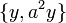# Element structure of direct product of D8 and Z2

Jump to: navigation, search

## Contents

This article gives specific information, namely, element structure, about a particular group, namely: direct product of D8 and Z2.
View element structure of particular groups | View other specific information about direct product of D8 and Z2

We use the following presentation, where$e$ denotes the identity element:$G := \langle a,x,y \mid a^4 = x^2 = y^2 = e, xax = a^{-1}, ya = ay, xy = yx \rangle$.

## Conjugacy class structure

FACTS TO CHECK AGAINST FOR CONJUGACY CLASS SIZES AND STRUCTURE:
Divisibility facts: size of conjugacy class divides order of group | size of conjugacy class divides index of center | size of conjugacy class equals index of centralizer
Bounding facts: size of conjugacy class is bounded by order of derived subgroup
Counting facts: number of conjugacy classes equals number of irreducible representations | class equation of a group
Conjugacy class Size of conjugacy class Order of elements in conjugacy class Centralizer of first element of class$\! \{ e \}$ 1 1 whole group$\! \{ a^2 \}$ 1 2 whole group$\! \{ y \}$ 1 2 whole group$\! \{ a^2y \}$ 1 2 whole group$\! \{ a, a^3 \}$ 2 4$\langle a, y \rangle$ -- isomorphic to direct product of Z4 and Z2$\! \{ ay, a^3y \}$ 2 4$\langle a, y \rangle$ -- isomorphic to direct product of Z4 and Z2$\! \{ x, a^2x \}$ 2 2$\langle a^2,x,y \rangle$ -- isomorphic to elementary abelian group:E8$\! \{ xy, a^2xy \}$ 2 2$\langle a^2, x, y\rangle$ -- isomorphic to elementary abelian group:E8$\! \{ ax, a^3x \}$ 2 2$\langle a^2, ax, y \rangle$ -- isomorphic to elementary abelian group:E8$\! \{ axy, a^3xy \}$ 2 2$\langle a^2, ax, y \rangle$ -- isomorphic to elementary abelian group:E8

The equivalence classes up to automorphisms are:

Equivalence class under automorphisms Size of equivalence class Number of conjugacy classes in it Size of each conjugacy class$\! \{ e \}$ 1 1 1$\! \{ a^2 \}$ 1 1 1$\! \{ y, a^2y \}$ 2 2 1$\! \{ a, a^3, ay, a^3y \}$ 4 2 2$\! \{ x, ax, a^2x, a^3x, xy, axy, a^2xy, a^3xy \}$ 8 4 2

## Order and power information

FACTS TO CHECK AGAINST:
1-ISOMORPHISM (cf. 1-isomorphic groups): Lazard Lie group is 1-isomorphic to the additive group of its Lazard Lie ring | order statistics-equivalent not implies 1-isomorphic

### Order statistics

To compare and contrast the order statistics of this group with other groups of the same order, refer element structure of groups of order 16#Order statistics
Number Elements of order exactly that number Number of such elements Number of conjugacy classes of such elements Number of elements whose order divides that number Number of conjugacy classes whose element order divides that number
1$\! \{ e \}$ 1 1 1 1
2$\! \{ a^2, y, a^2y, x, ax, a^2x, a^3x, xy, axy, a^2xy, a^3xy \}$ 11 7 12 8
4$\! \{ a, a^3, ay, a^3y \}$ 4 2 16 10

### Power statistics

Number$d$$d^{th}$ powers that are not$k^{th}$ powers for any larger divisor$k$ of the group order Number of such elements Number of conjugacy classes of such elements Number of$d^{th}$ powers Number of conjugacy classes of$d^{th}$ powers
1$\! \{ y, a^2y, x, ax, a^2x, a^3x, xy, axy, a^2xy, a^3xy, a, a^3, ay, a^3y \}$ 14 8 16 10
2$\! \{ a^2 \}$ 1 1 2 2
4 -- 0 0 1 1
8 -- 0 0 1 1
16$\! \{ e \}$ 1 1 1 1